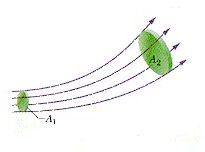# Fluid Particles, Streamtubes and Derivation of Continuity Equation

For the analysis of elastic bodies we divide it smaller elements and then analyze each element and their interaction to obtain the understanding of the dynamics of the complete body. Similarly we divide fluid flow in smaller or simpler parts such that each can be analyzed easily, and then the solution from each part is integrated to arrive at an overall picture of the fluid flow.

## Fluid Particles

Fluid flow is nowhere close to the motion of a rigid body but it can be divided into small parts, Fluid Particles, which can be treated as rigid bodies. Basic Newton’s laws of motion for particles can be applied to these fluid particles. Equation for fluid flow can be written as for the system of particles. This definition of fluid flow is helpful where these particles can be tracked and are identifiable.

## Streamtubes

The flow element which is bounded by streamlines is called as a Streamtube. The size of streamtubes can vary, they can be defined in different sizes, and the only constraint is that the bounding surface of a Streamtube should be made up of streamlines. As the name denotes, it’s a tube, which indicates that flow is possible only through the tube and not across the tube. This is because of the way in which a Streamtube is defined.The streamtube is bounded by streamlines and the velocity of flow at any point on a streamline is tangential to that streamline. So, there is no possibility of any flow across the surface of a streamtube. Thus, a streamtube behaves as a tube indeed. The definition of the streamtube is particularly useful for deriving a continuity equation for fluid flow.

For deriving the continuity equation, consider an elemental streamtube. Since there is no mass flow across the surface of the streamtube, by the law of conservation of mass, we can say mass entering the streamtube per second is equal to the mass leaving the tube per second. This can be integrated and written for a finite streamtube with average values at the entrance and exit of the streamtube. For incompressible steady flow the equation for conservation of mass is reduced to the continuity equation.

## This post is part of the series: Fundamentals of Fluid Flow

How does a flowing fluid behaves,and how do the different parameters vary in a flowing fluid? Fluid flow analysis involves theoretical and experimental study of the flow of fluid by defining flow models and observation of variation of parameters. This series will discuss Fundamentals of Fluid Flow.